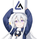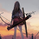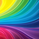1216浏览
The first edition；

This is a tool to calculate BTC long “Margin Call” price line, you can intuitively adjust the leverage multiple to calculate the current “Margin Call” price line position, you can also adjust the average price to see the average“Margin Call” price；

## 评论Pretty cool, i was curious about the indicator so ii would have been great to have more information about it. Note that you can calculate the extremities the with fewer operations using
```ma = sma(src,maval)
ma1 = ma*xa/(0.995*xa + 1)
ma2 = ma*xb/(0.995*xb + 1)
ma3 = ma*xc/(0.995*xc + 1)
ma4 = ma*xd/(0.995*xd + 1)
ma5 = ma*xe/(0.995*xe + 1)```

Looking forward to your other indicators.alexgrover
@alexgrover,I am a newbie; thanks for your tips; Thanks a lot；Thank you kindly for publishing this indicator as well as sharing the pine script code :)
I like how it works as support and resistance where at times the EMA ribbon does not
awesome idea
I would like this indicator twice if I could !